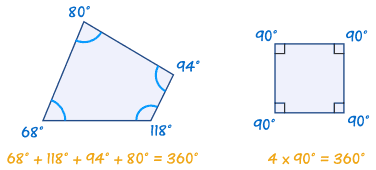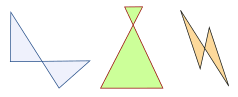## Monday, May 4, 2020

(quad means four, lateral means side).
A Quadrilateral has four-sides, it is 2-dimensional (a flat shape), closed (the lines join up), and has straight sides.
• four sides (edges)
• four vertices (corners)
• interior angles that add to 360 degrees:• Try drawing a quadrilateral, and measure the angles. They should add to 360°

There are special types of quadrilateral:Some types are also included in the definition of other types! For example a squarerhombus and rectangle are also parallelogramsSee below for more details.
Let us look at each type in turn:

## The Rectanglethe little squares in each corner mean "right angle"
rectangle is a four-sided shape where every angle is a right angle (90°).
Also opposite sides are parallel and of equal length.

## The Squarethe little squares in each corner mean "right angle"
square has equal sides (marked "s") and every angle is a right angle (90°)
Also opposite sides are parallel.
A square also fits the definition of a rectangle (all angles are 90°), and a rhombus (all sides are equal length).

## The Rhombusrhombus is a four-sided shape where all sides have equal length (marked "s").
Also opposite sides are parallel and opposite angles are equal.Another interesting thing is that the diagonals (dashed lines) meet in the middle at a right angle. In other words they "bisect" (cut in half) each other at right angles.
A rhombus is sometimes called a rhomb or a diamond.

## The Parallelogramparallelogram has opposite sides parallel and equal in length. Also opposite angles are equal (angles "A" are the same, and angles "B" are the same).
NOTE: Squares, Rectangles and Rhombuses are all Parallelograms!

## The KiteHey, it looks like a kite (usually).
It has two pairs of sides:
Each pair is made of two equal-length sides that join up.
Also:
• the angles where the two pairs meet are equal.
• the diagonals, shown as dashed lines above, meet at a right angle.
• one of the diagonals bisects (cuts equally in half) the other.

... and that's it for the special quadrilaterals.

Using the chart below we can answer such questions as:
• Is a Square a type of Rectangle? (Yes)
• Is a Rectangle a type of Kite? (No)Oh Yes! when two sides cross over, we call it a "Complex" or "Self-Intersecting" quadrilateral, like these:They still have 4 sides, but two sides cross over.

## Polygon

A quadrilateral is a polygon. In fact it is a 4-sided polygon, just like a triangle is a 3-sided polygon, a pentagon is a 5-sided polygon, and so on.
Disclaimer: We use this Video OR any content for Non-Profit ,provided by this is meant for Free Education purpose ONLY